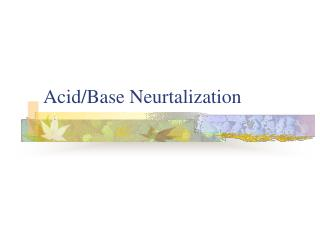DownloadDownload PresentationAcid/Base Neurtalization

# Acid/Base Neurtalization

Télécharger la présentation## Acid/Base Neurtalization

- - - - - - - - - - - - - - - - - - - - - - - - - - - E N D - - - - - - - - - - - - - - - - - - - - - - - - - - -
##### Presentation Transcript

1. Acid/Base Neurtalization

2. Acid-Base Reactions • Acid – substance that increases the H+ concentration in aqueous solutions • HCl → H+ + Cl- • H2SO4→ H+ + HSO4- • HSO4→ H+ + SO42-

3. Base • Increases the OH- concentration • NaOH → Na + OH- • NH3 + H2O → NH4+ + OH-

4. Neutralization • Acids and bases react together to produce a salt (ionic compound) and water • HCl + NaOH → NaCl + H2O • Write a balanced equation for the reaction of hydrobromic acid, HBr, with barium hydroxide, Ba(OH)2.

5. Example • Write the reaction that occurs when hydrochloric acid is mixed with silver hydroxide

6. Write the balanced equation for the reaction between phosphoric acid and potassium hydroxide.

7. Examples • Aqueous acetic aceticacid is mixed with aqueous potassium hydroxide. Write the equation for the reaction.

8. Example • What volume of a .100M HCl solution is needed to neutralize 25.0 ml of .350M NaOH?

9. Example • In a reaction 28.0 ml of .250M HNO3 and 53.0 ml of .320M NaOH are mixed. Calculate the the amount of water formed.